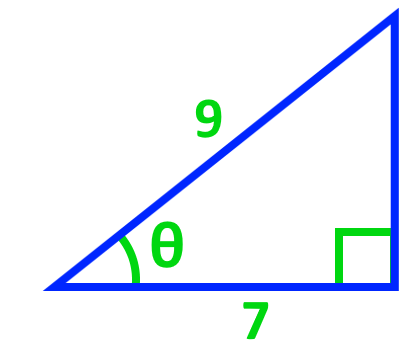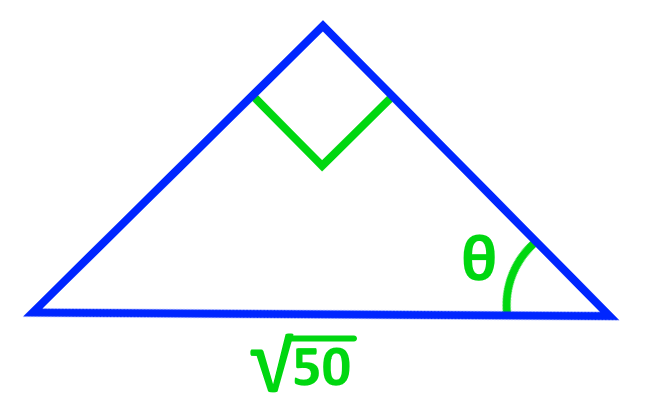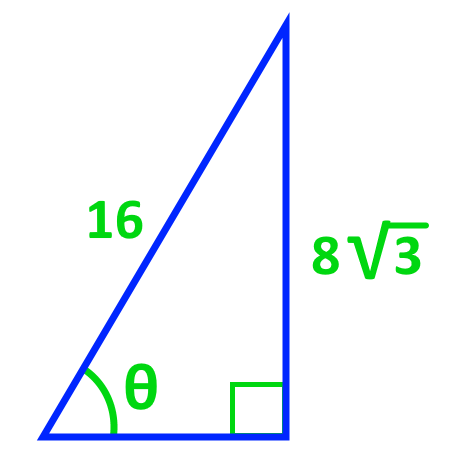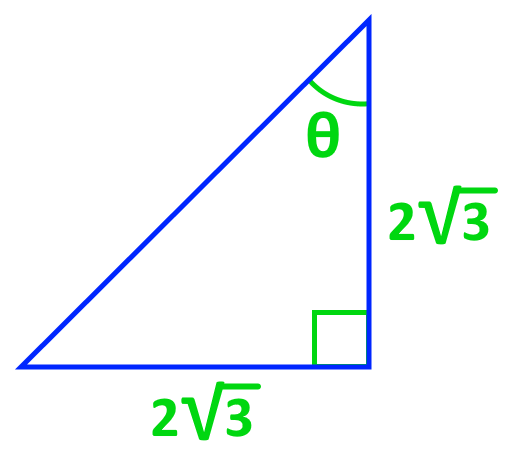# Evaluating inverse trigonometric functions#### Everything You Need in One Place

Homework problems? Exam preparation? Trying to grasp a concept or just brushing up the basics? Our extensive help & practice library have got you covered.#### Learn and Practice With Ease

Our proven video lessons ease you through problems quickly, and you get tonnes of friendly practice on questions that trip students up on tests and finals.#### Instant and Unlimited Help

Our personalized learning platform enables you to instantly find the exact walkthrough to your specific type of question. Activate unlimited help now!##### Intros
###### Lessons
1. Application of the Cancellation Laws

Introduction to Evaluating Inverse Trigonometric Functions

##### Examples
###### Lessons
1. Understanding the Use of Inverse Trigonometric Functions

Find the angles for each of the following diagrams.

1.2.2. Find the angle for the following isosceles triangle.1. Determining the Angles in Exact Values by Using Special Triangles

Find the angles for each of the following diagrams in exact value.

1.2.2. Application of the Cancellation Laws

Solve the following inverse trigonometric functions:

1. $\sin (\sin^{-1} 0.5)$
2. $\cos^{-1} (\cos \frac{\pi}{4})$
3. $\sin^{-1} (\sin \frac{3\pi}{4})$
3. Solving Expressions With One Inverse Trigonometry

Solve the following inverse trigonometric functions:

1. $\cos^{-1} \frac{1}{2}$
2. $\sin^{-1} \frac{1}{2}$
4. Evaluating Expressions With a Combination of Inverse and Non-Inverse Trigonometry

Solve the following inverse trigonometric functions:

1. $\sin (\cos^{-1} \frac{\sqrt 3}{2})$
2. $\cos (\sin^{-1} \frac{2}{3})$
3. $\cos (2\tan^{-1} \sqrt 2)$
4. $\cos (\sin^{-1} x)$
5. Special Cases: Evaluating Functions With Numbers Outside of the Restrictions

Solve the following inverse trigonometric functions:

1. $\cos^{-1} (\cos \frac{3\pi}{2})$
2. $\sin^{-1} (\sin \frac{5\pi}{2})$
###### Topic Notes

In this lesson, we will learn:

• Application of the Cancellation Laws
• Solving Expressions With One Inverse Trigonometry
• Evaluating Expressions With a Combination of Inverse and Non-Inverse Trigonometry
• Special Cases: Evaluating Functions With Numbers Outside of the Restrictions

Cancellation Laws:

$\sin^{-1} (\sin x) = x\;$, $-\frac{\pi}{2} \leq x \leq \frac{\pi}{2}$

$\sin (\sin^{-1} x) = x\;$, $-1 \leq x \leq 1$

$\cos^{-1} (\cos x) = x\;$, $0 \leq x \leq \pi$

$\cos (\cos^{-1} x) = x\;$, $-1 \leq x \leq 1$

$\tan^{-1} (\tan x) = x\;$, $-\frac{\pi}{2} \leq x \leq \frac{\pi}{2}$

$\tan (\tan^{-1} x) = x\;$, $-\infty$ < $x$ < $\infty$

Trigonometric Identity:

$\cos 2\theta = \cos^{2} \theta - \sin^{2} \theta$